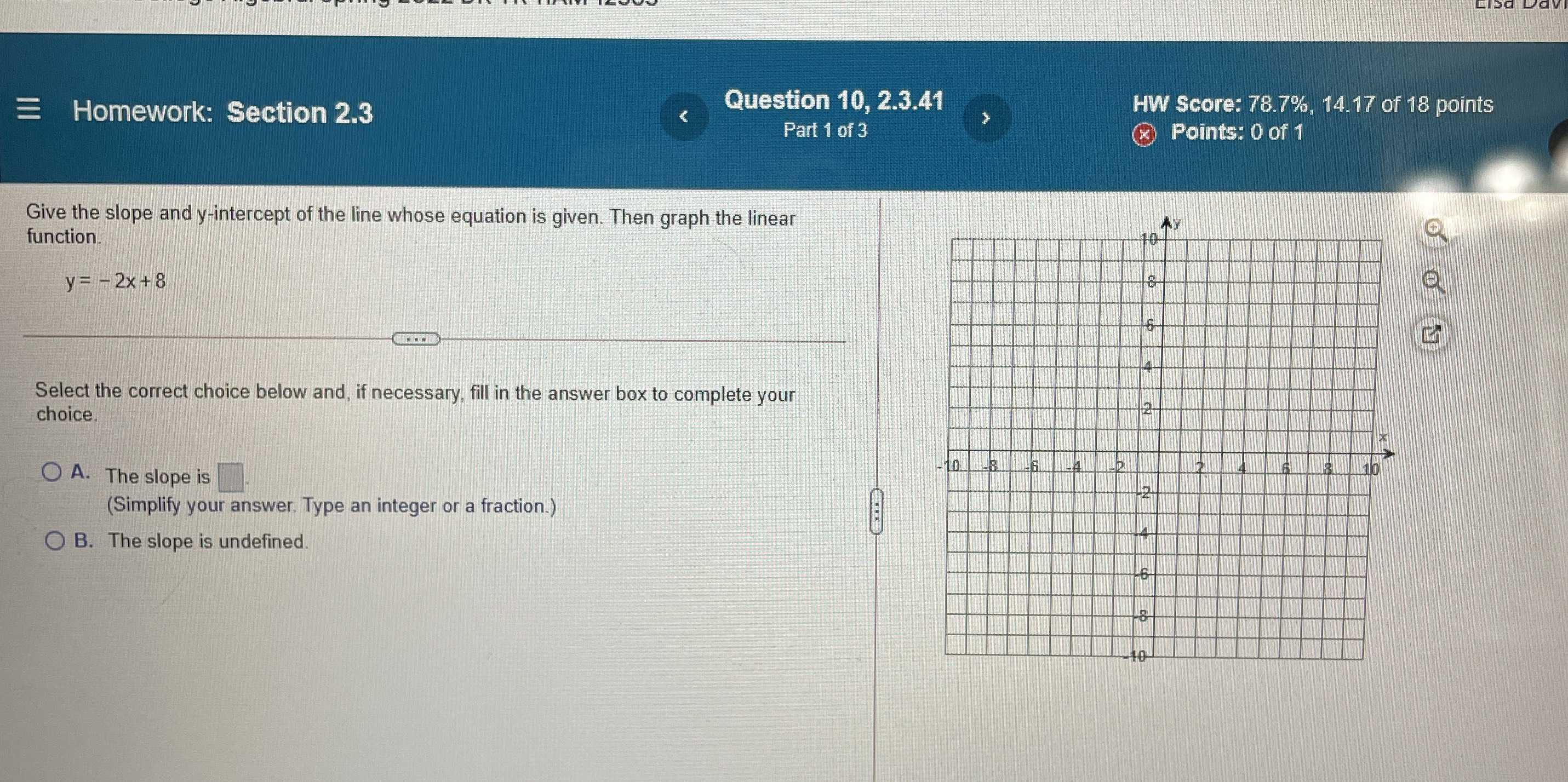### ¿Todavía tienes preguntas de matemáticas?

Pregunte a nuestros tutores expertos
Algebra
PreguntaGive the slope and $$y$$ -intercept of the line whose equation is given. Then graph the linear function. $$y = - 2 x + 8$$Select the correct choice below and, if necessary, fill in the answer box to complete your choice. A. The slope is (Simplify your answer. Type an integer or a fraction.) B. The slope is undefined.

$$A.- 2$$